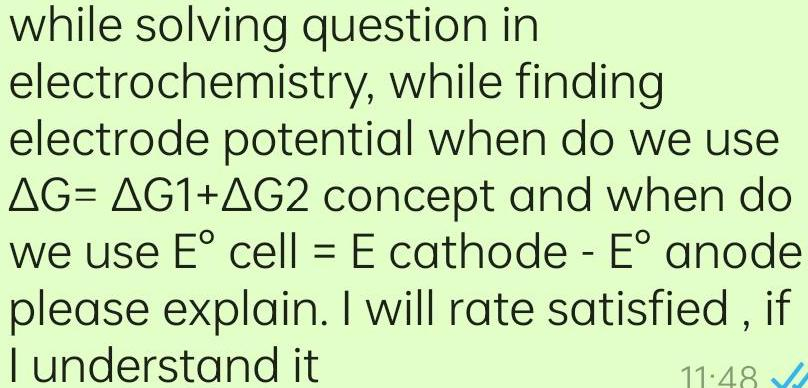Physical Chemistry
Electrochemistry
while solving question in electrochemistry while finding electrode potential when do we use AG AG1 AG2 concept and when do we use E cell E cathode E anode please explain I will rate satisfied if I understand it 11 48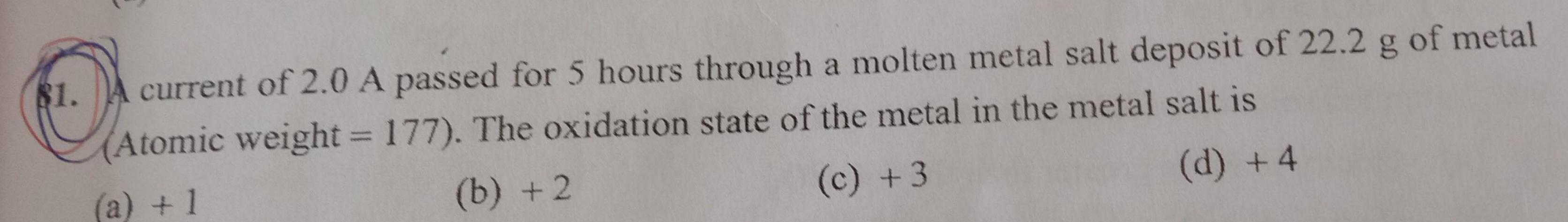Physical Chemistry
Electrochemistry
81 A current of 2 0 A passed for 5 hours through a molten metal salt deposit of 22 2 g of metal Atomic weight 177 The oxidation state of the metal in the metal salt is a 1 b 2 c 3 d 4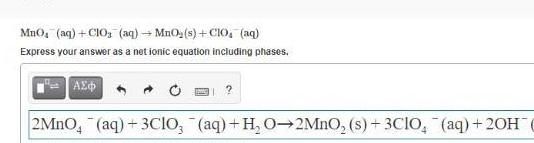Physical Chemistry
Electrochemistry
MnO aq CIO aq MnO s C10 aq Express your answer as a net ionic equation including phases AE 2MnO4 aq 3CIO aq H O 2MnO s 3ClO aq 20H7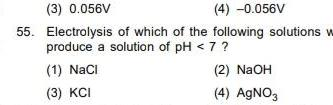Physical Chemistry
Electrochemistry
3 0 056V 4 0 056V 55 Electrolysis of which of the following solutions w produce a solution of pH 7 2 NaOH 4 AgNO3 1 NaCl 3 KCI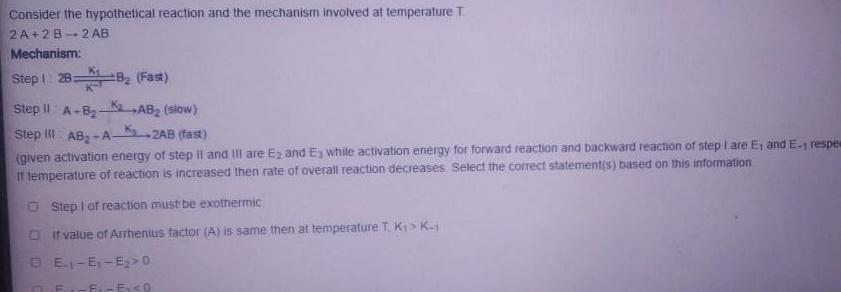Physical Chemistry
Electrochemistry
Consider the hypothetical reaction and the mechanism involved at temperature T 2A 2B 2 AB Mechanism Step 1 28 Step II A B Step III AB A 2AB fast given activation energy of step II and III are E and E while activation energy for forward reaction and backward reaction of step I are E and E 1 respe If temperature of reaction is increased then rate of overall reaction decreases Select the correct statement s based on this information AB slow K Step 1 of reaction must be exothermic DIf value of Arrhenius factor A is same then at temperature T K K 1 DE E E O F F Ex 0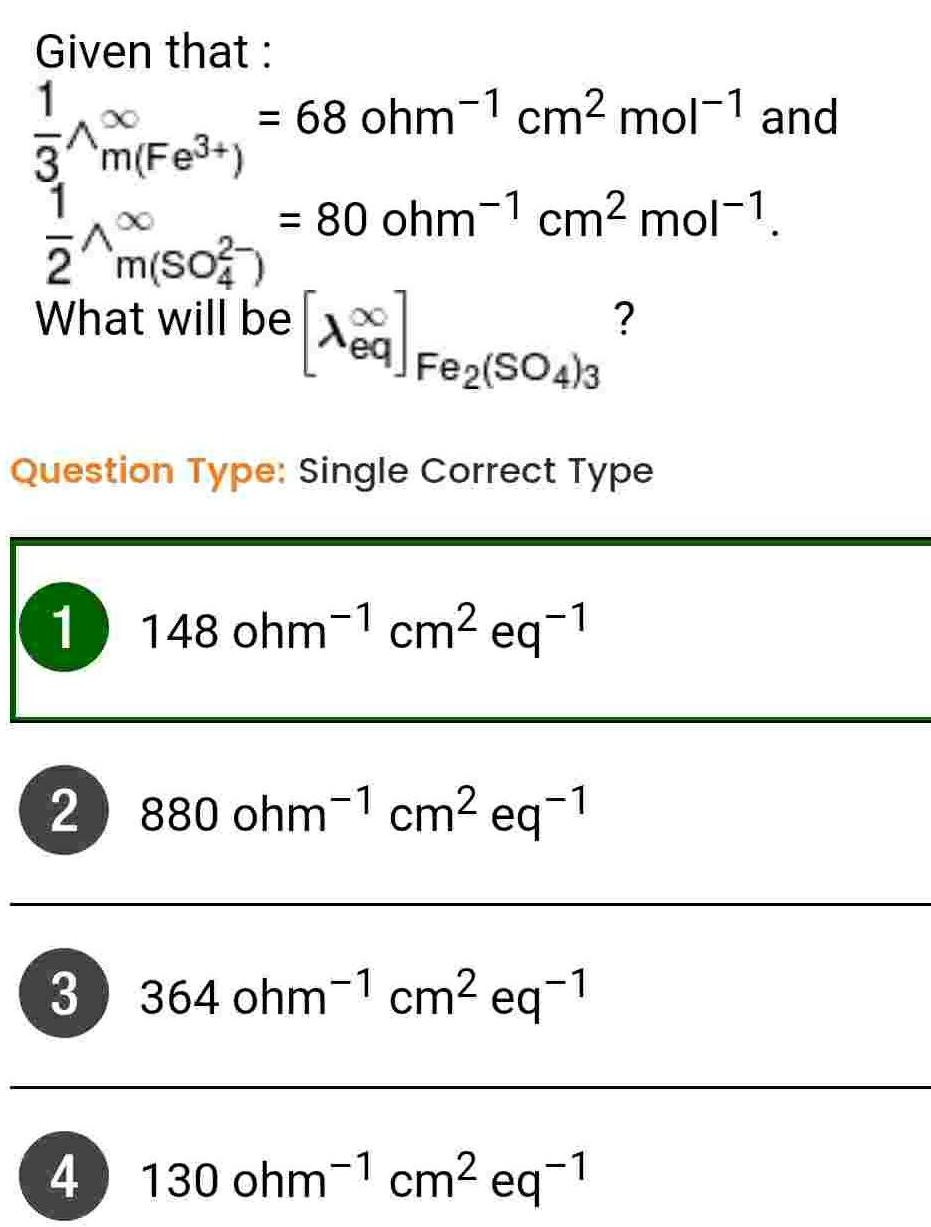Physical Chemistry
Electrochemistry
Given that 1 3 m Fe Z m 50 What will be 169 Fe2 SO4 3 eq Question Type Single Correct Type 2 68 ohm 1 cm mol 1 and 1 148 ohm 1 cm eq 1 3 80 ohm cm mol 1 4 880 ohm 1 cm eq 1 364 ohm 1 cm eq 1 130 ohm 1 cm eq 1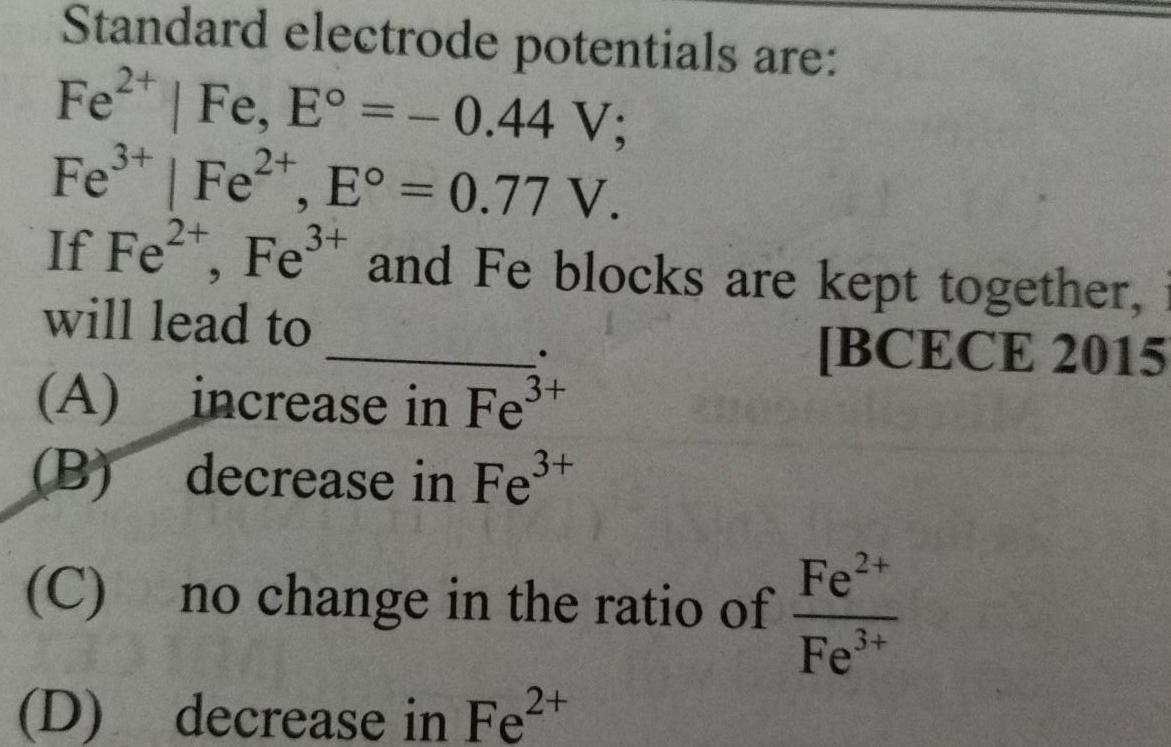Physical Chemistry
Electrochemistry
Standard electrode potentials are Fe Fe E 0 44 V 3 Fe Fe E 0 77 V 3 If Fe Fe and Fe blocks are kept together will lead to BCECE 2015 A B C 3 increase in Fe decrease in Fe no change in the ratio of D decrease in Fe Fe Fe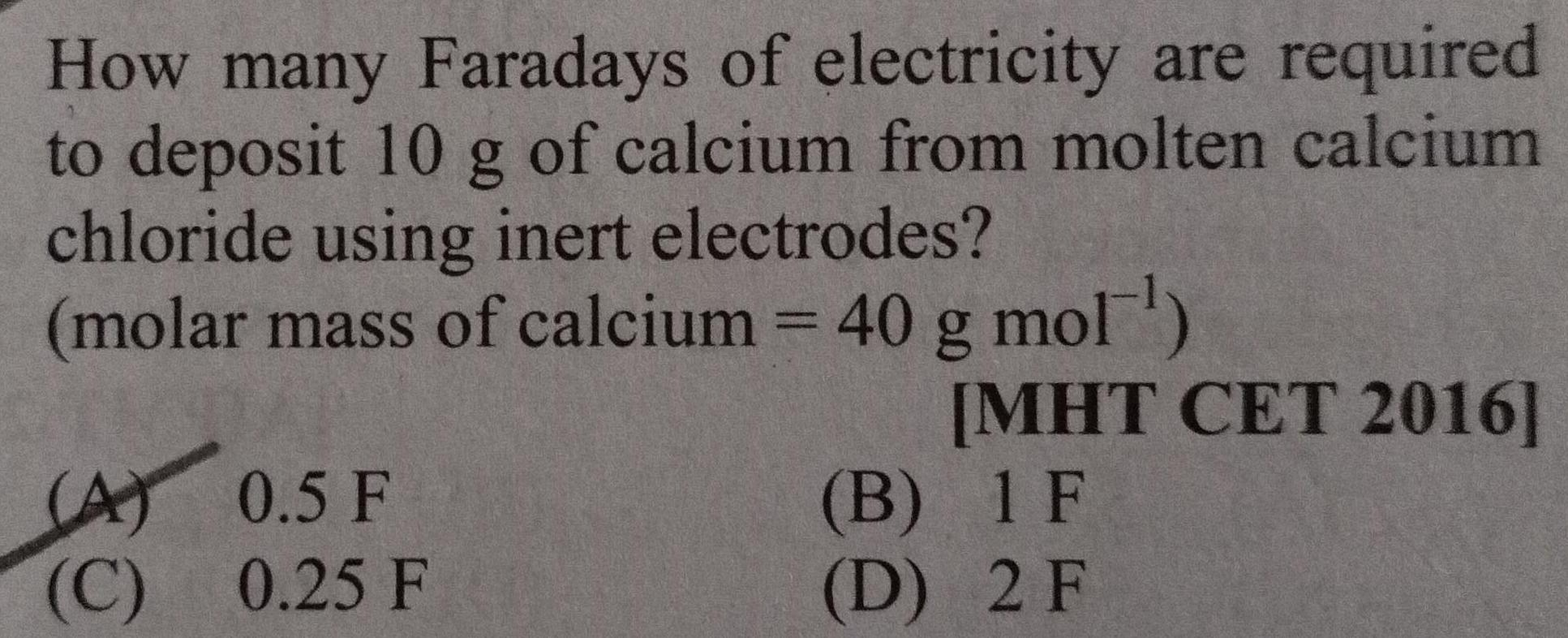Physical Chemistry
Electrochemistry
How many Faradays of electricity are required to deposit 10 g of calcium from molten calcium chloride using inert electrodes molar mass of calcium 40 g mol A 0 5 F C 0 25 F B D MHT CET 2016 1 F 2 F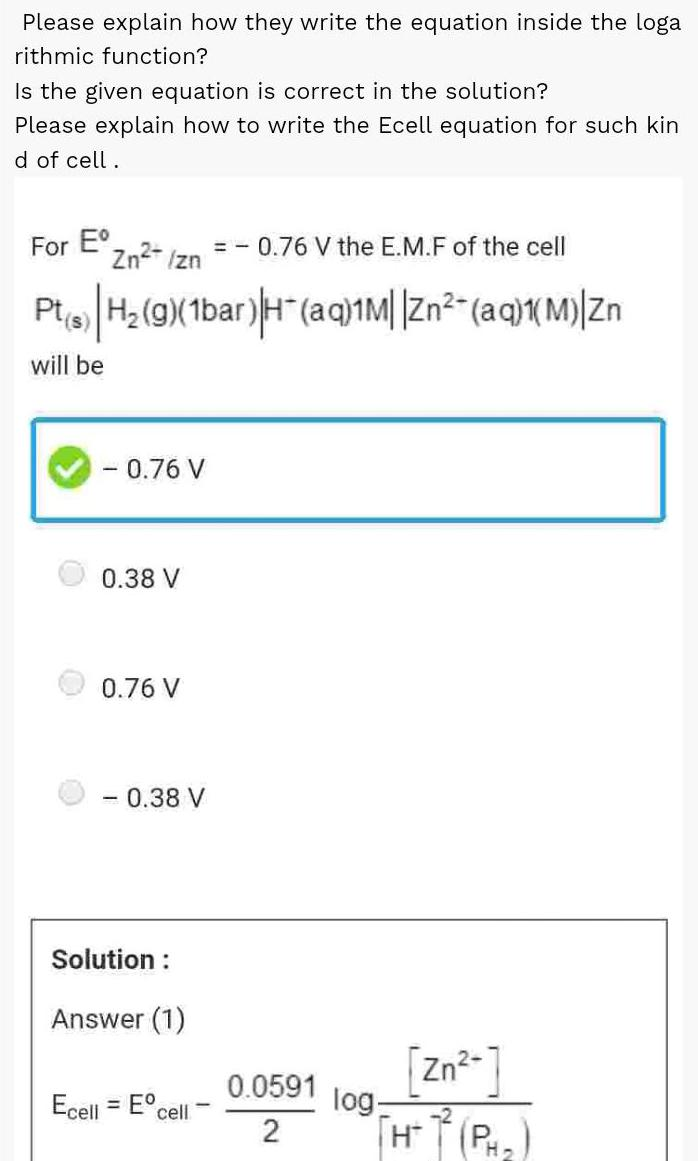Physical Chemistry
Electrochemistry
Please explain how they write the equation inside the loga rithmic function Is the given equation is correct in the solution Please explain how to write the Ecell equation for such kin d of cell For E 0 76 V the E M F of the cell Pt s H g 1bar H aq 1M Zn aq 1 M Zn will be Zn zn 0 76 V 0 38 V Ecell 0 76 V 0 38 V Solution Answer 1 F cell 0 0591 2 Zn THTP log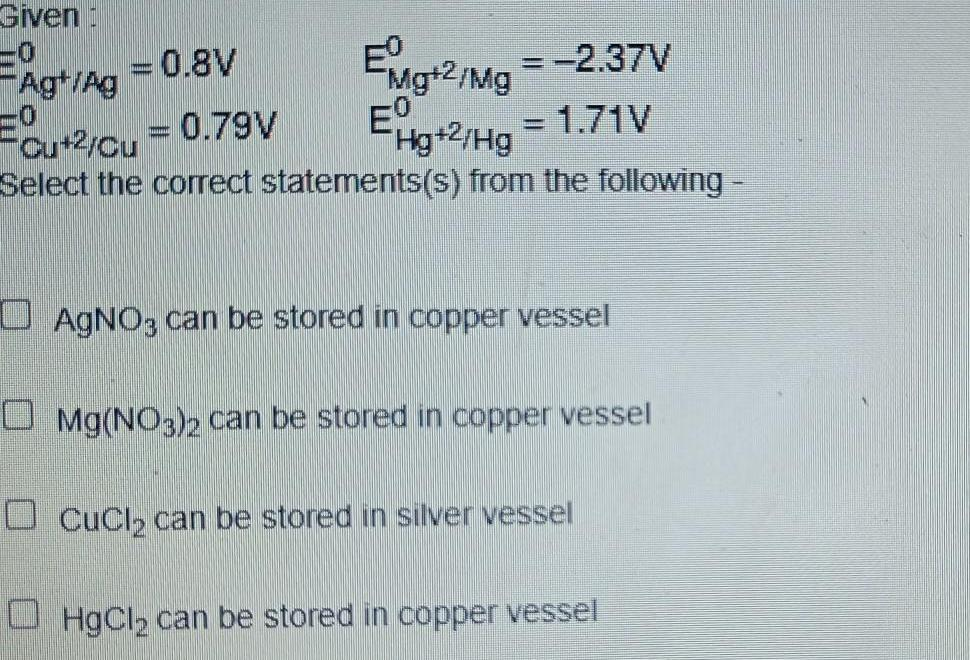Physical Chemistry
Electrochemistry
Given 0 Mg 2 Mg EHg 2 Hg 2 37V 1 71V husnail 0 8V Ag Ag 0 79V Cu 2 Cu Select the correct statements s from the following AgNO3 can be stored in copper vessel Mg NO3 2 can be stored in copper vessel CuCl can be stored in silver vessel HgCl can be stored in copper vessel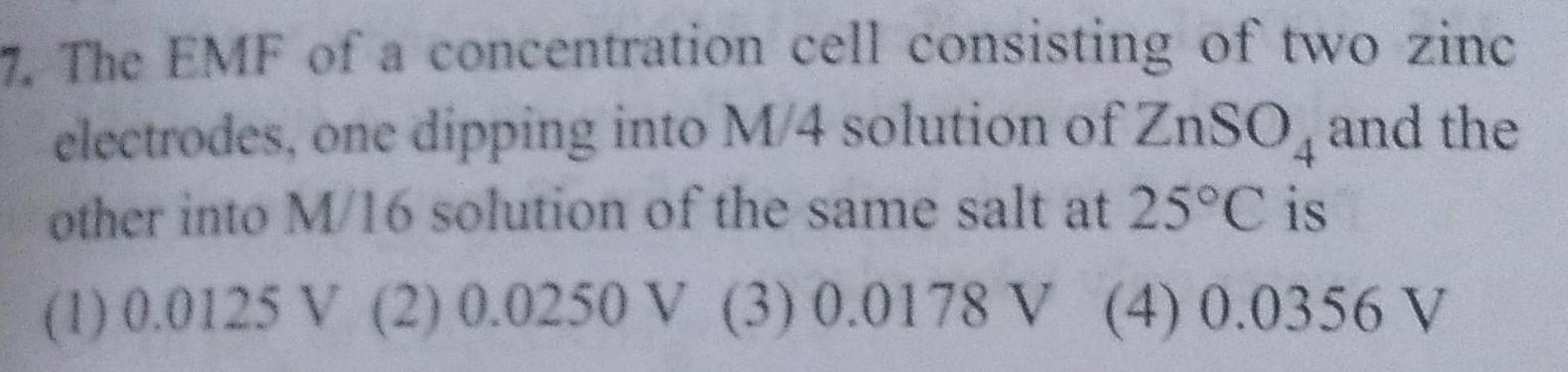Physical Chemistry
Electrochemistry
7 The EMF of a concentration cell consisting of two zinc electrodes one dipping into M 4 solution of ZnSO and the other into M 16 solution of the same salt at 25 C is 1 0 0125 V 2 0 0250 V 3 0 0178 V 4 0 0356 V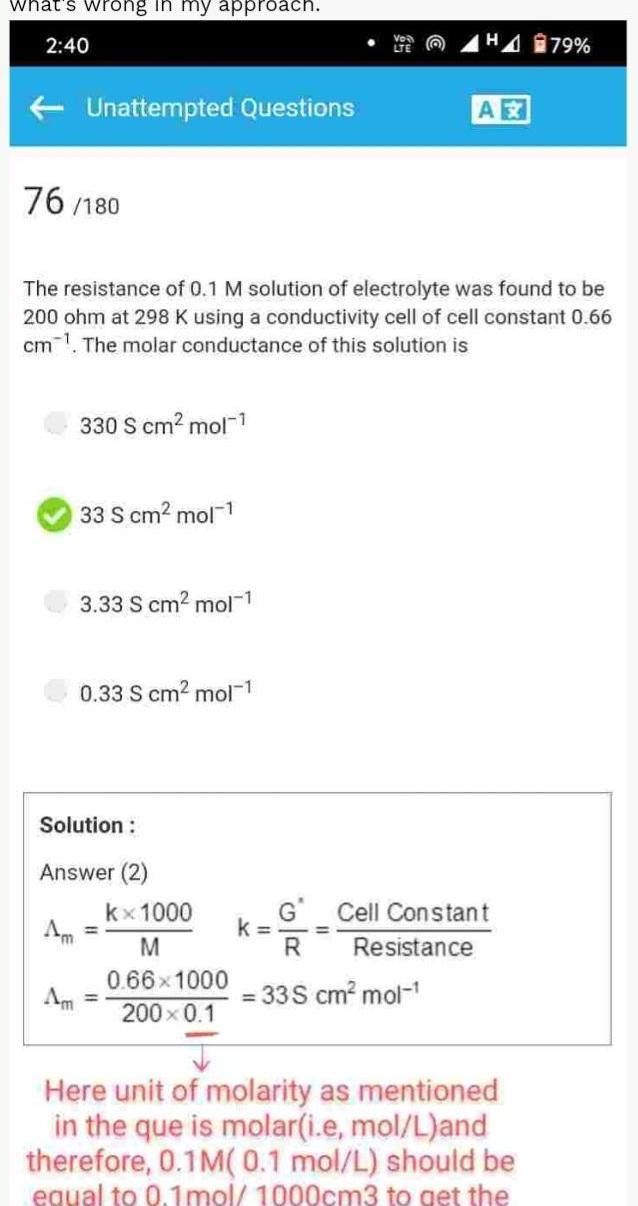Physical Chemistry
Electrochemistry
what s wrong in my approach 2 40 Unattempted Questions 76 180 330 S cm mol The resistance of 0 1 M solution of electrolyte was found to be 200 ohm at 298 K using a conductivity cell of cell constant 0 66 cm The molar conductance of this solution is 33 S cm mol 1 3 33 S cm mol 1 0 33 S cm mol 1 Solution Answer 2 kx 1000 M 0 66 1000 200 0 1 k G HA 79 A R 33S cm mol Cell Constant Resistance Here unit of molarity as mentioned in the que is molar i e mol L and therefore 0 1M 0 1 mol L should be equal to 0 1mol 1000cm3 to get the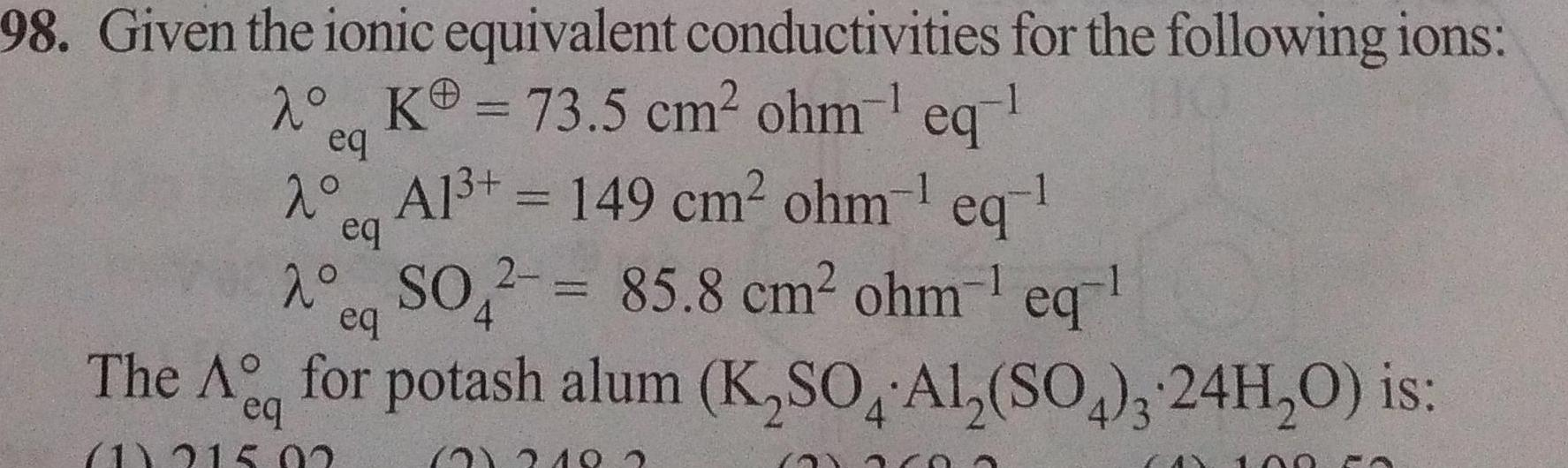Physical Chemistry
Electrochemistry
98 Given the ionic equivalent conductivities for the following ions K 73 5 cm ohm eq 2 eq 2 Al 149 cm ohm eq eq ASO2 85 8 cm ohm eq eq 4 The A for potash alum K SO AL SO 24H O is 4 eq 1 215 02 2 219 4 100 PA A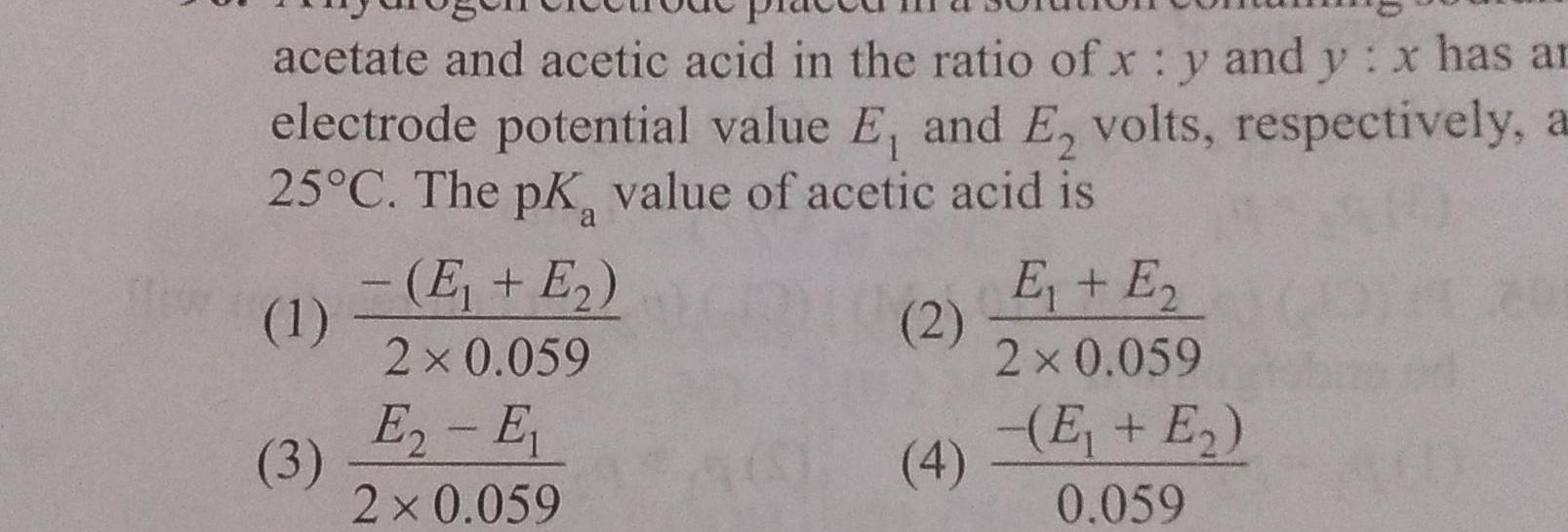Physical Chemistry
Electrochemistry
acetate and acetic acid in the ratio of x y and y x has ar electrode potential value E and E volts respectively a 25 C The pK value of acetic acid is 1 3 E E 2 0 059 E E 2 0 059 2 4 E E 2 0 059 E E 0 059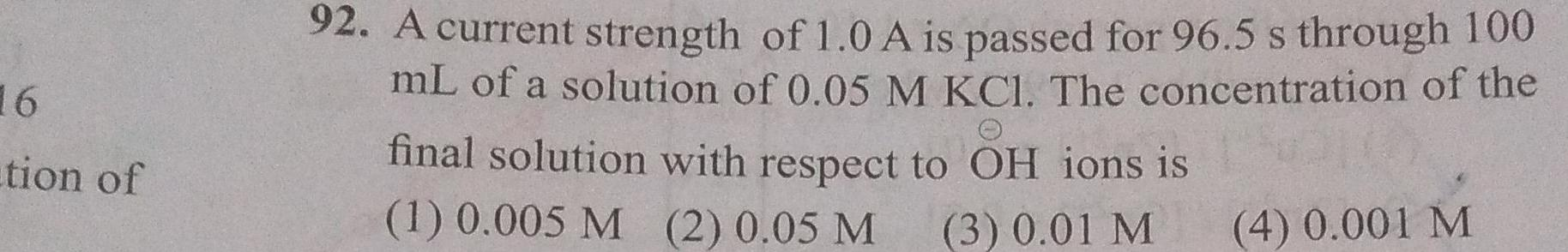Physical Chemistry
Electrochemistry
16 tion of 92 A current strength of 1 0 A is passed for 96 5 s through 100 mL of a solution of 0 05 M KCl The concentration of the final solution with respect to OH ions is 1 0 005 M 2 0 05 M 3 0 01 M 4 0 001 M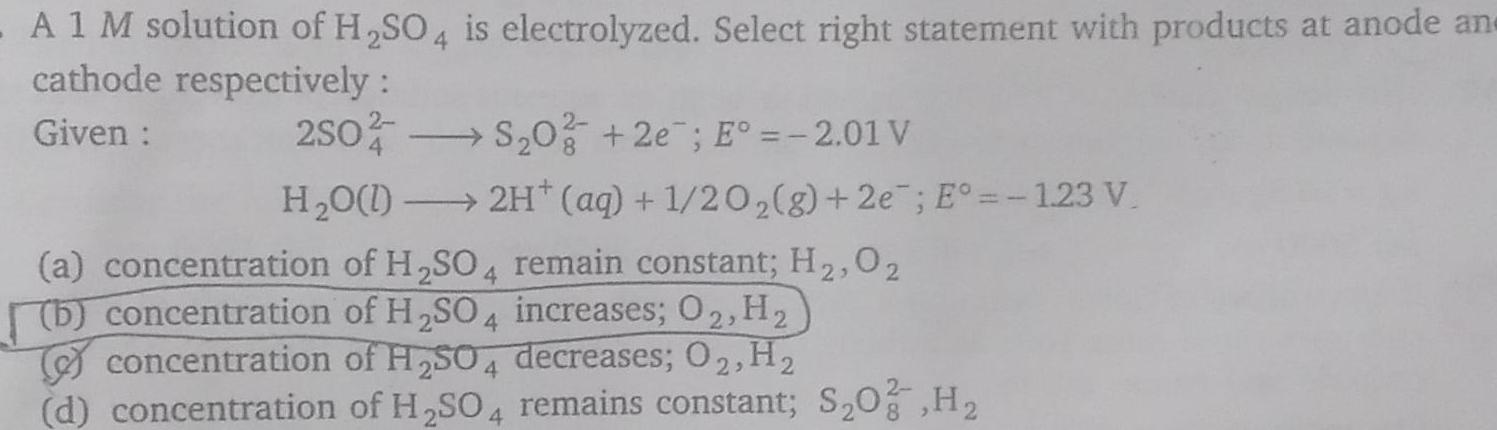Physical Chemistry
Electrochemistry
A 1 M solution of H SO4 is electrolyzed Select right statement with products at anode an cathode respectively Given 2SO4 S 0 2e E 2 01 V H O l 2H aq 1 20 2 g 2e E 1 23 V a concentration of H SO4 remain constant H 02 b concentration of H SO4 increases O2 H concentration of H SO4 decreases O2 H d concentration of H SO4 remains constant S O2 H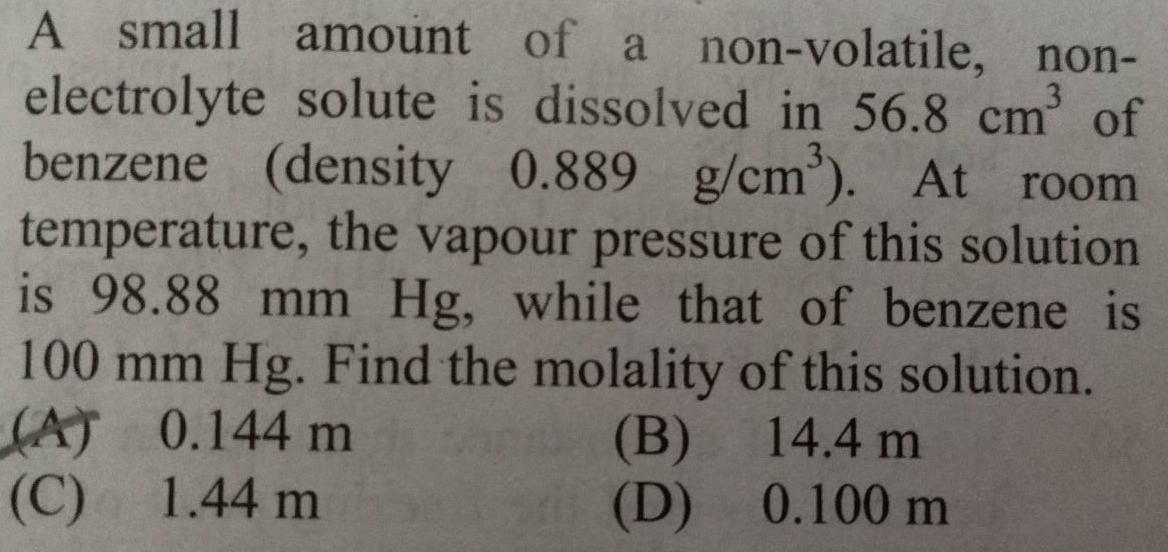Physical Chemistry
Electrochemistry
A small amount of a non volatile non electrolyte solute is dissolved in 56 8 cm of benzene density 0 889 g cm At room temperature the vapour pressure of this solution is 98 88 mm Hg while that of benzene is 100 mm Hg Find the molality of this solution A 0 144 m 14 4 m C 1 44 m 0 100 m B D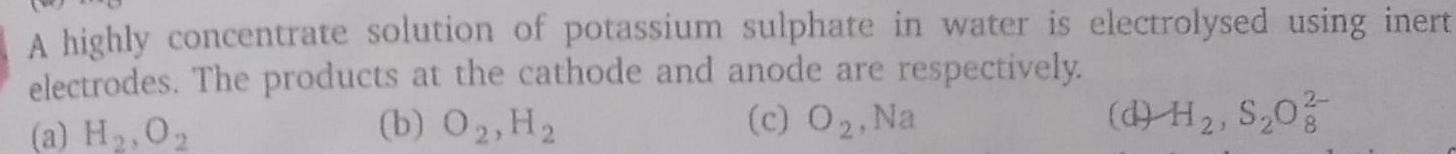Physical Chemistry
Electrochemistry
A highly concentrate solution of potassium sulphate in water is electrolysed using inert electrodes The products at the cathode and anode are respectively a H 0 2 b O2 H c 0 Na d H S 0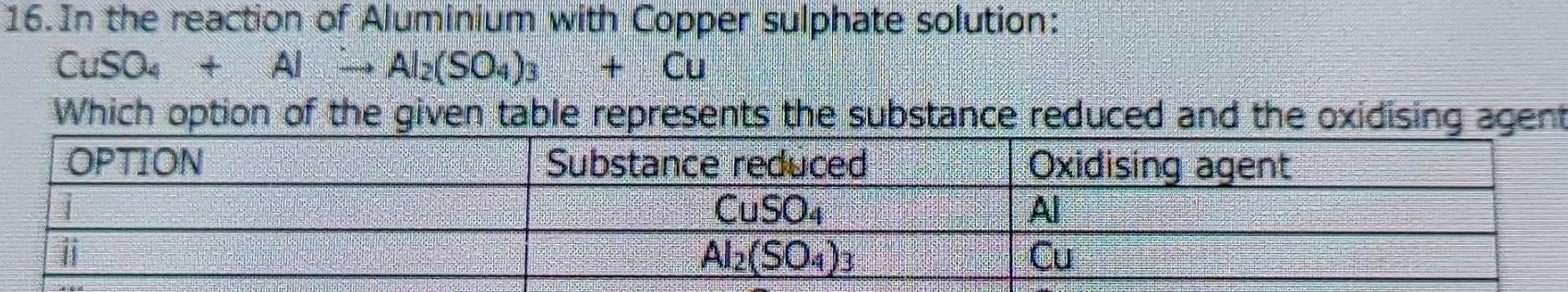Physical Chemistry
Electrochemistry
16 In the reaction of Aluminium with Copper sulphate solution CuSO4 Al Al2 SO4 3 Which option of the given table represents the substance reduced and the oxidising agent OPTION Oxidising agent Substance reduced CuSO4 Al2 SO4 3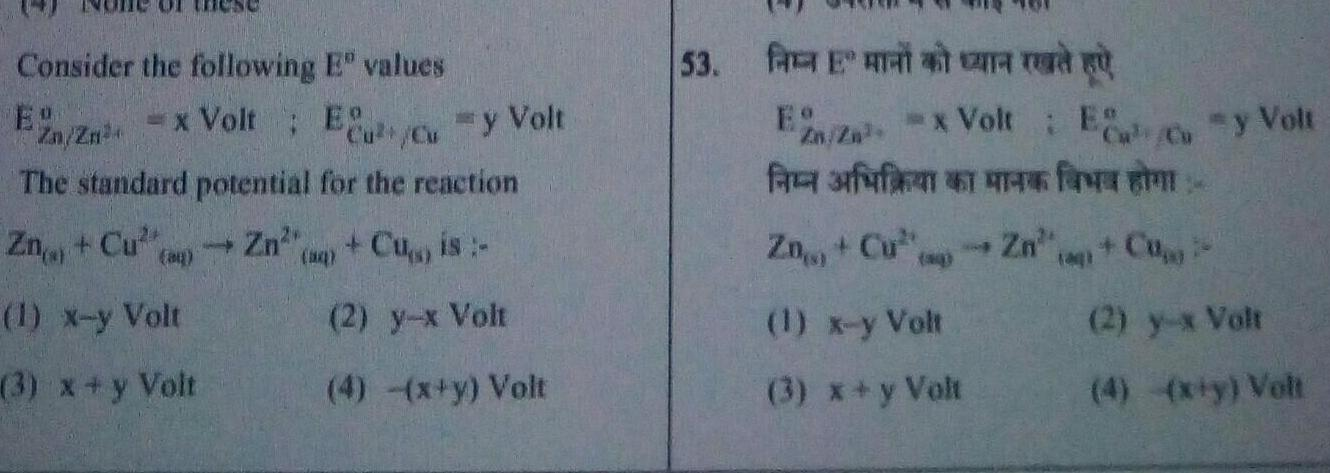Physical Chemistry
Electrochemistry
Consider the following E values x Volt Eu Cu y Volt Zn Zn 4 The standard potential for the reaction Zn Cu Zn Cu is ay x 2 y x Volt 4 x y Volt 1 x y Volt 3 x y Volt 53 En g x Volt E Affin Cu Zn 20 1 x y Volt 3 x y Volt Zn y Volt Co 2 y x Volt 4 x y Volt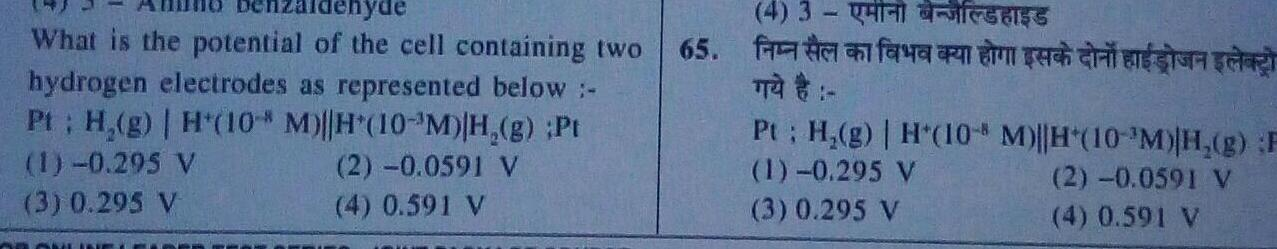Physical Chemistry
Electrochemistry
What is the potential of the cell containing two hydrogen electrodes as represented below Pt H g H 10 M H 10 M H g Pt 1 0 295 V 3 0 295 V 2 0 0591 V 4 0 591 V 65 4 3 TT Pt H g H 10 1 0 295 V 3 0 295 V TOASORT M H 10 M H g F 2 0 0591 V 4 0 591 V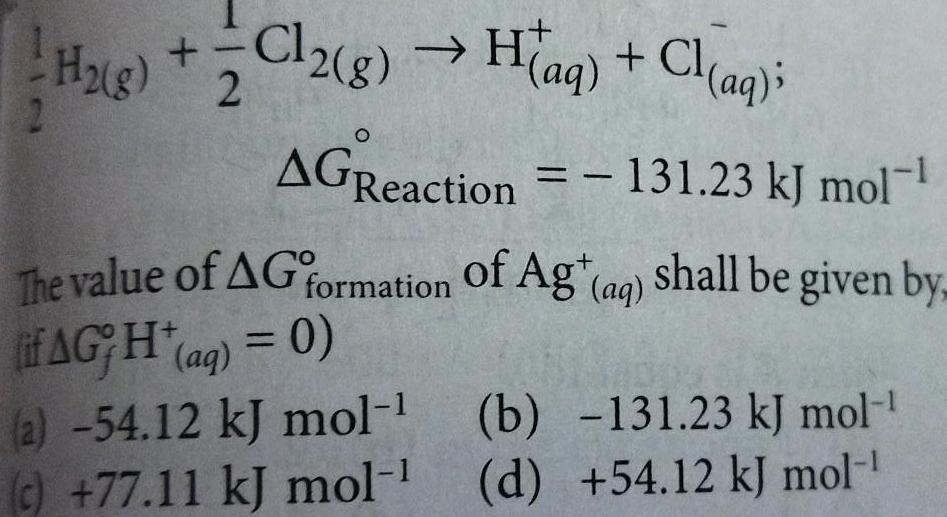Physical Chemistry
Electrochemistry
H g 2 Cl2 g H aq Cl aq AGReaction 131 23 kJ mol The value of AG formation of Ag aq of if AG H aq 0 Agt aq shall be given by a 54 12 kJ mol b c 77 11 kJ mol d 131 23 kJ mol 54 12 kJ mol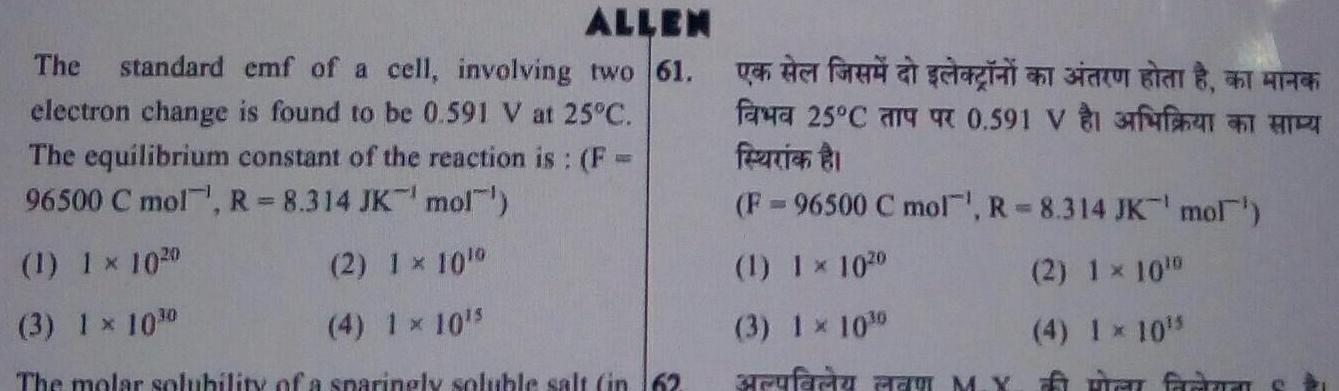Physical Chemistry
Electrochemistry
ALLEN The standard emf of a cell involving two 61 electron change is found to be 0 591 V at 25 C The equilibrium constant of the reaction is F 96500 C mol R 8 314 JK mol 1 1 1020 2 1 10 3 1 1030 4 1 10 5 The molar solubility of a sparingly soluble salt in 162 var for seg i 25 591 frenian F 96500 C mol R 8 314 JK mol 1 1 1020 3 1 10 erufaru a 2 1 10 0 4 1 10 few framan s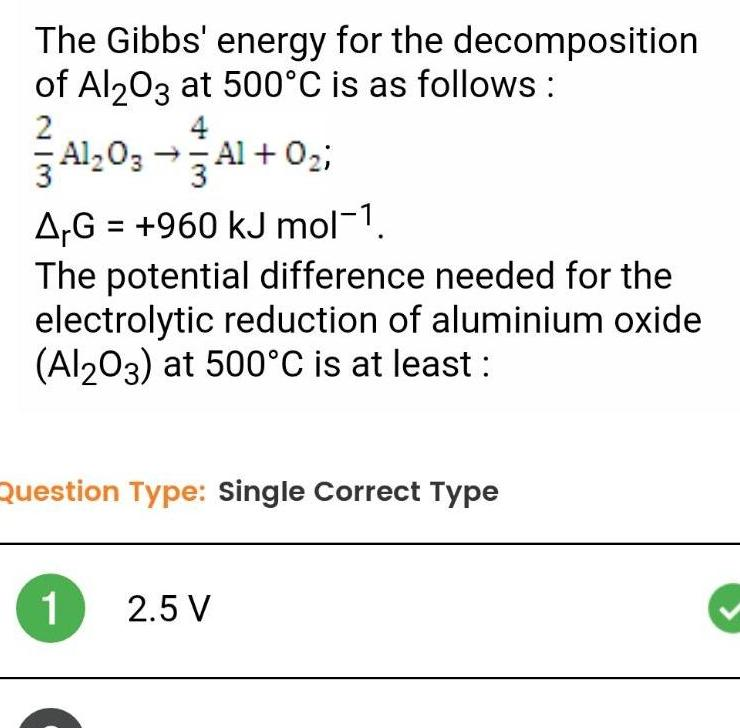Physical Chemistry
Electrochemistry
The Gibbs energy for the decomposition of Al2O3 at 500 C is as follows 2 4 Al2O3 Al O ArG 960 kJ mol 1 The potential difference needed for the electrolytic reduction of aluminium oxide Al2O3 at 500 C is at least Question Type Single Correct Type 1 2 5 V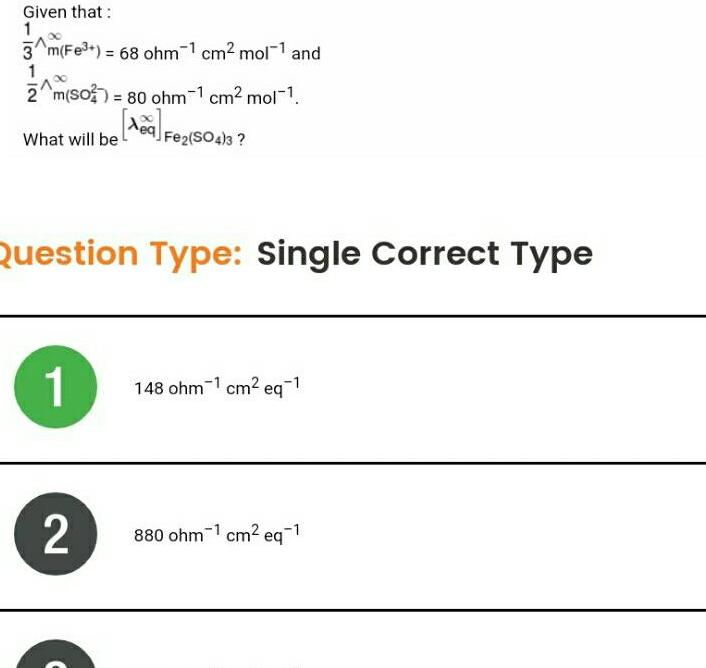Physical Chemistry
Electrochemistry
Given that 1 3 m Fe 68 ohm 1 cm mol 1 and 1 2 m SO2 80 ohm 1 cm mol 1 What will be Fe2 SO4 3 Question Type Single Correct Type 1 2 148 ohm1 cm eq 1 880 ohm cm eq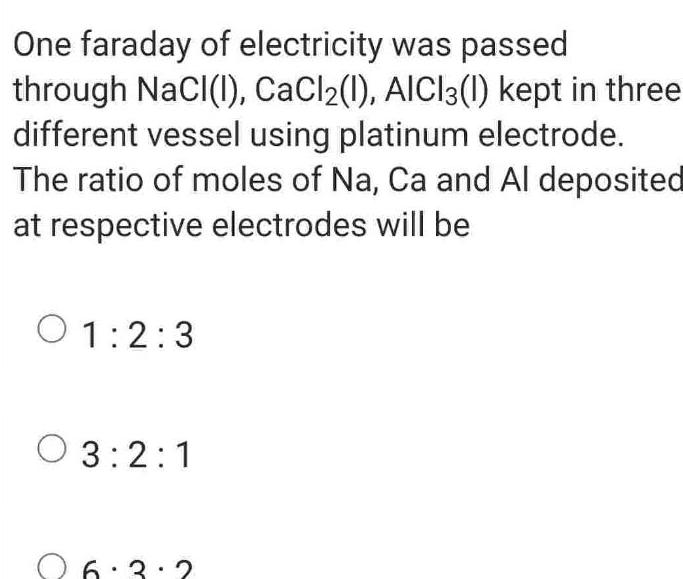Physical Chemistry
Electrochemistry
One faraday of electricity was passed through NaCl 1 CaCl2 1 AlCl3 1 kept in three different vessel using platinum electrode The ratio of moles of Na Ca and Al deposited at respective electrodes will be 01 2 3 03 2 1 26 3 2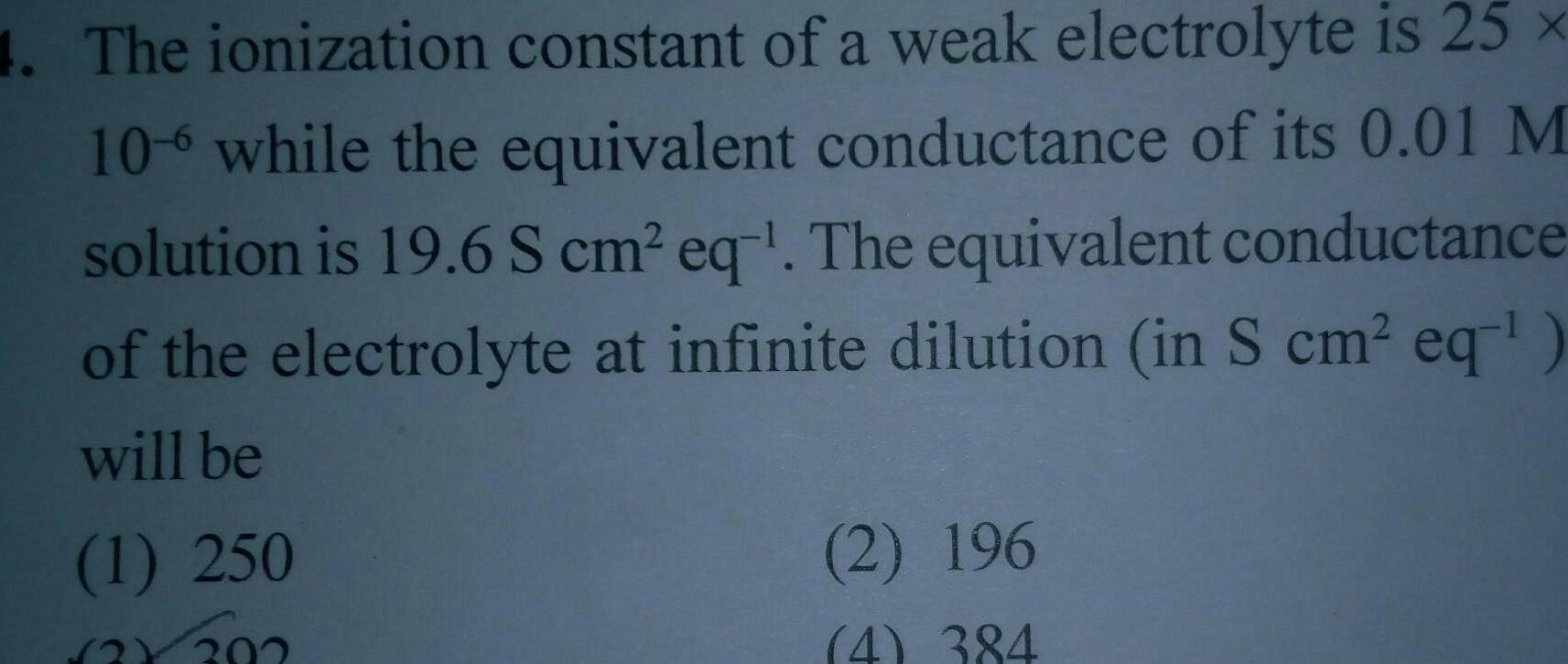Physical Chemistry
Electrochemistry
4 The ionization constant of a weak electrolyte is 25 x 106 while the equivalent conductance of its 0 01 M solution is 19 6 S cm eq The equivalent conductance of the electrolyte at infinite dilution in S cm eq will be 1 250 2X202 2 196 4 384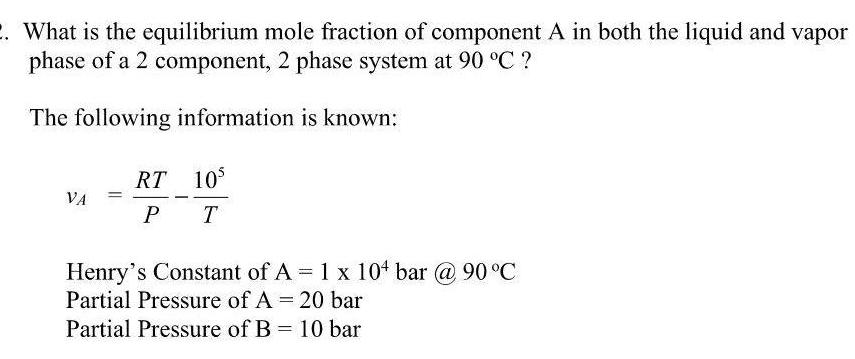Physical Chemistry
Electrochemistry
2 What is the equilibrium mole fraction of component A in both the liquid and vapor phase of a 2 component 2 phase system at 90 C The following information is known VA RT 10 PT Henry s Constant of A 1 x 10 bar 90 C Partial Pressure of A 20 bar Partial Pressure of B 10 barPhysical Chemistry
Electrochemistry
ALLEN 1000 mL 1 M CuSO aq is electrolysed by 53 9 65 amp current for 100 sec using Pt electrode Which is incorrect statemenet 1 Blue colour intensity decreases during electrolysis 2 Blue colour intensity remains constant if Cu electrode used 3 pH of solution is 8 after electrolysis 4 At anode O gas liberated during electrolysis Which of the fo 1000 ff 1 M CuSO aq 9 65 A 100 g 1 2 Cu n fa feer Tel 8 1 4 440 324 pH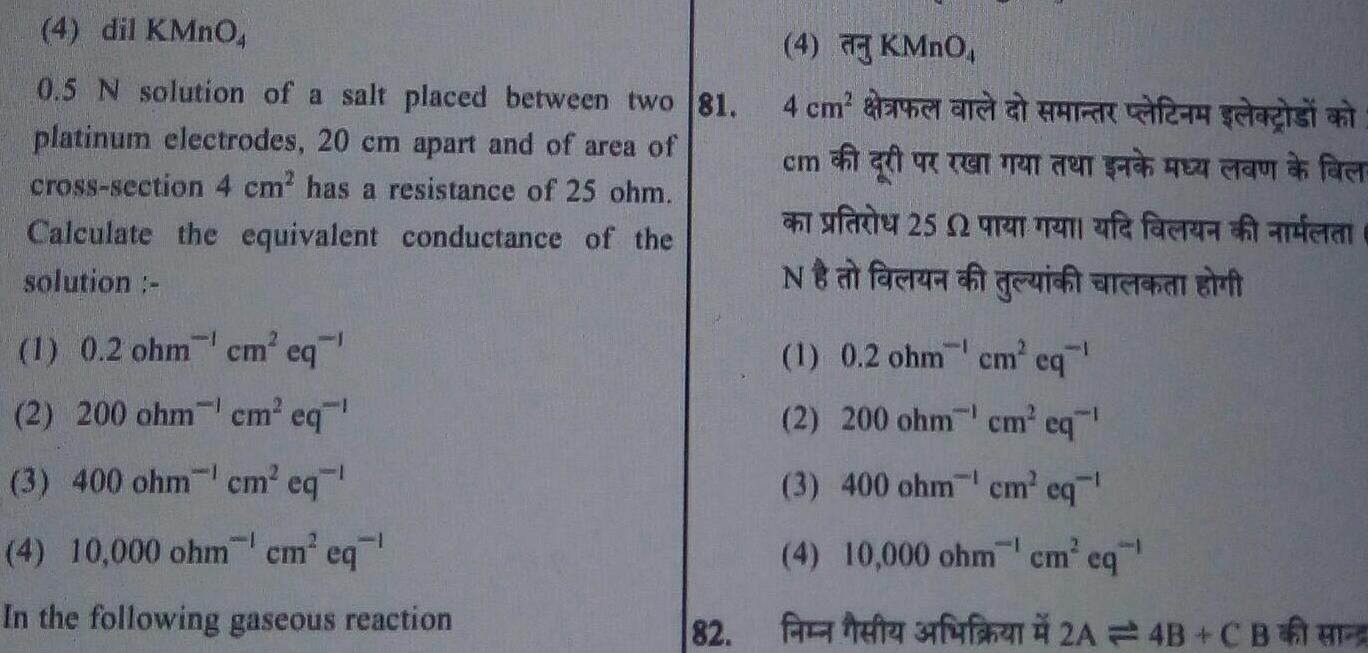Physical Chemistry
Electrochemistry
4 dil KMnO4 0 5 N solution of a salt placed between two 81 platinum electrodes 20 cm apart and of area of cross section 4 cm has a resistance of 25 ohm Calculate the equivalent conductance of the solution 1 1 0 2 ohm cm eq 2 200 ohm cm eq 3 400 ohm cm eq 4 10 000 ohmcm eq In the following gaseous reaction 82 4 KMnO 4 cm men HR f gist and den farer 25 fe facrari guit Na faca 1 0 2 ohm cm eq 1 2 200 ohm cm eq 3 400 ohm cm eq 4 10 000 ohm cm eq fa tefta fufa 2A4B C BE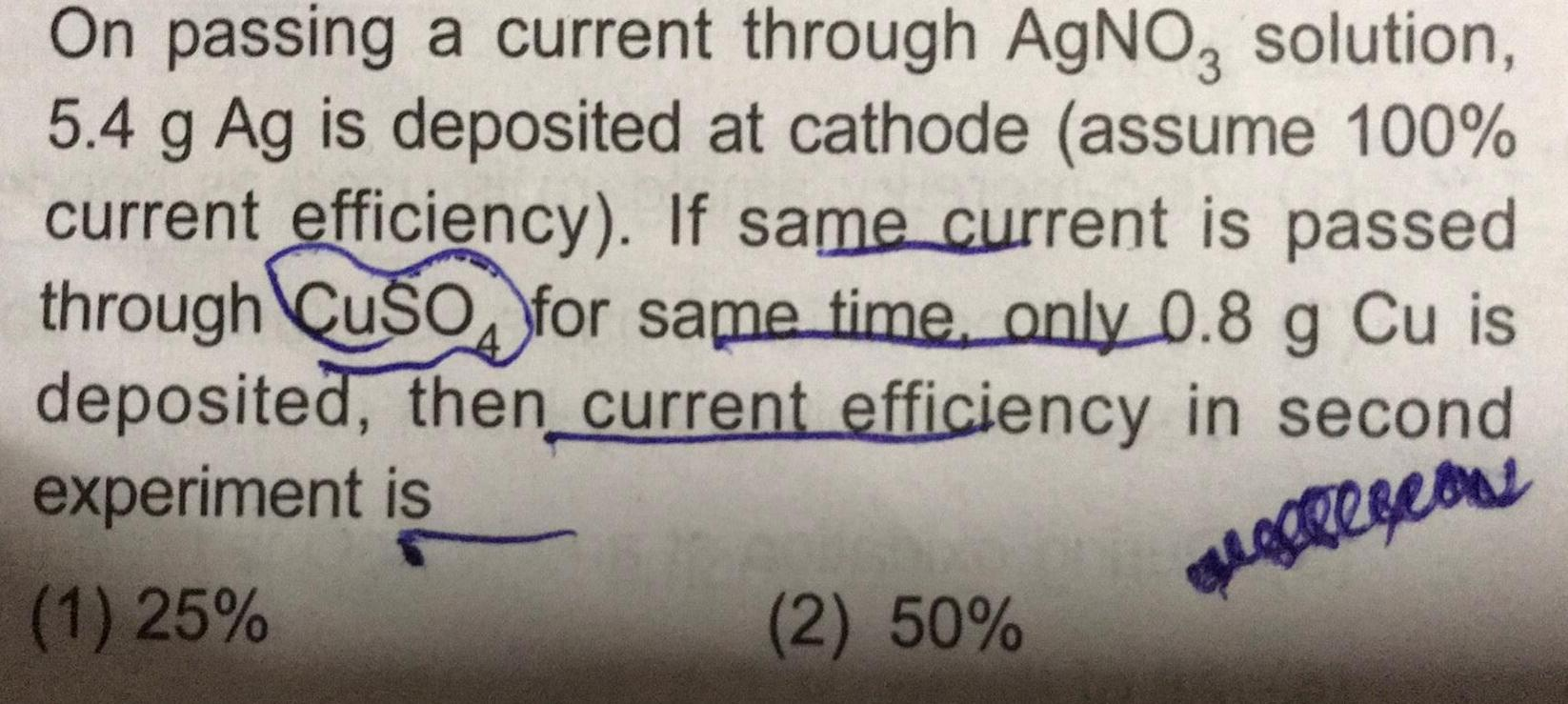Physical Chemistry
Electrochemistry
On passing a current through AgNO3 solution 5 4 g Ag is deposited at cathode assume 100 current efficiency If same current is passed through CuSO for same time only 0 8 g Cu is deposited then current efficiency in second experiment is 1 25 leon 2 50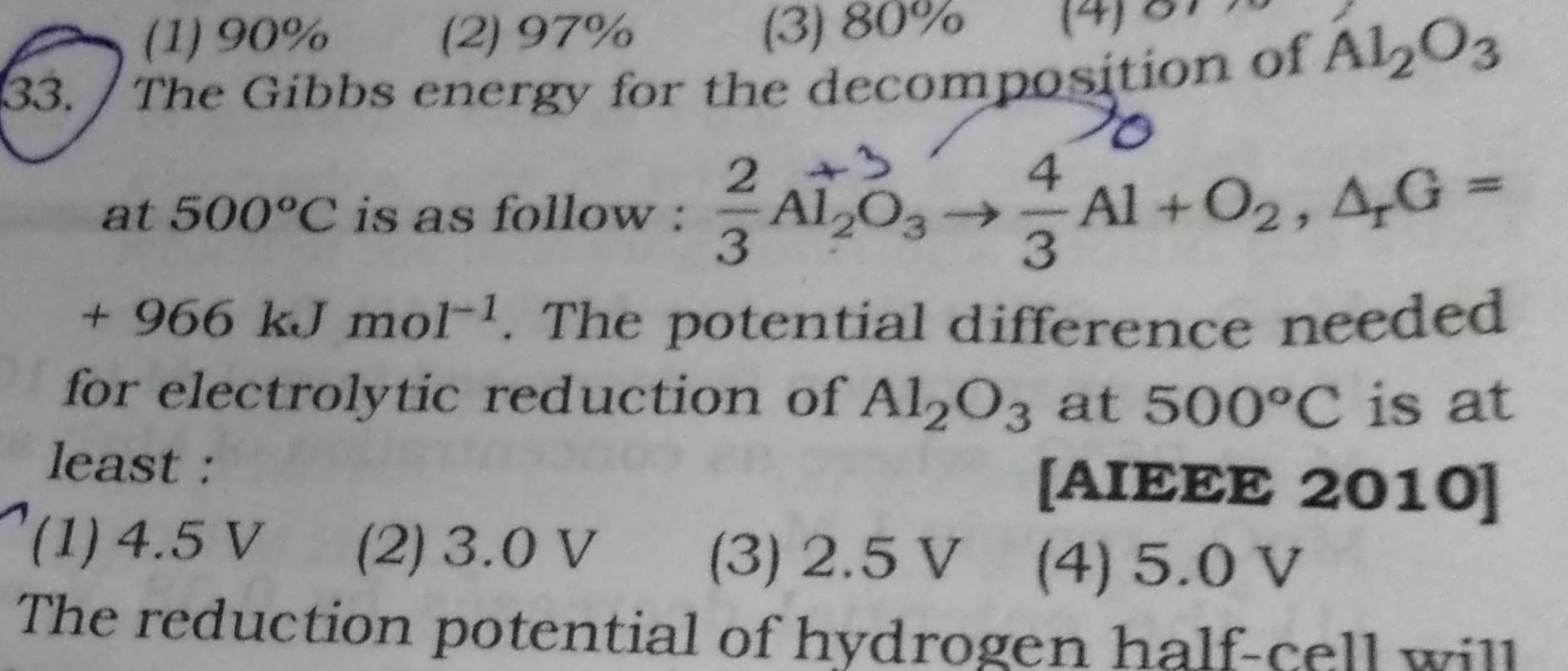Physical Chemistry
Electrochemistry
33 1 90 2 97 3 80 The Gibbs energy for the decomposition of Al2O3 2 3 4 3 3 at 500 C is as follow Al2O3 Al O 4G 966 kJ mol The potential difference needed for electrolytic reduction of Al2O3 at 500 C is at least AIEEE 2010 1 4 5 V 2 3 0 V 3 2 5 V 4 5 0 V The reduction potential of hydrogen half cell will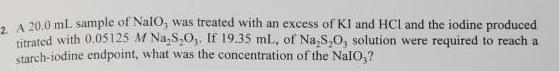Physical Chemistry
Electrochemistry
2 A 20 0 mL sample of Nalo was treated with an excess of KI and HCl and the iodine produced titrated with 0 05125 M Na S O If 19 35 mL of Na S O solution were required to reach a starch iodine endpoint what was the concentration of the NalO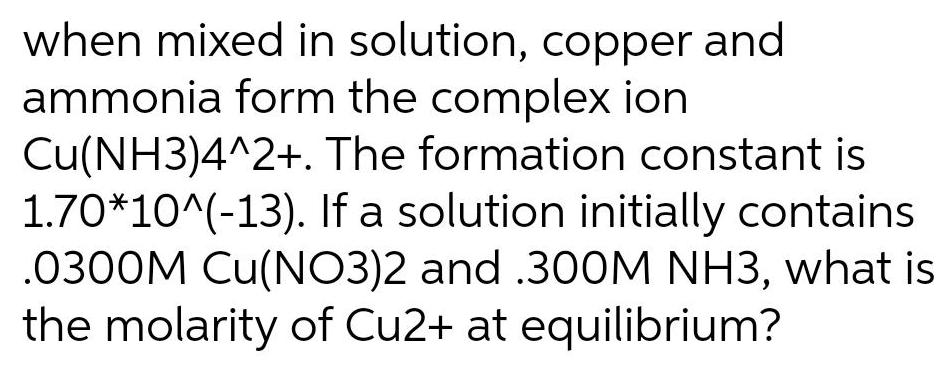Physical Chemistry
Electrochemistry
when mixed in solution copper and ammonia form the complex ion Cu NH3 4 2 The formation constant is 1 70 10 13 If a solution initially contains 0300M Cu NO3 2 and 300M NH3 what is the molarity of Cu2 at equilibrium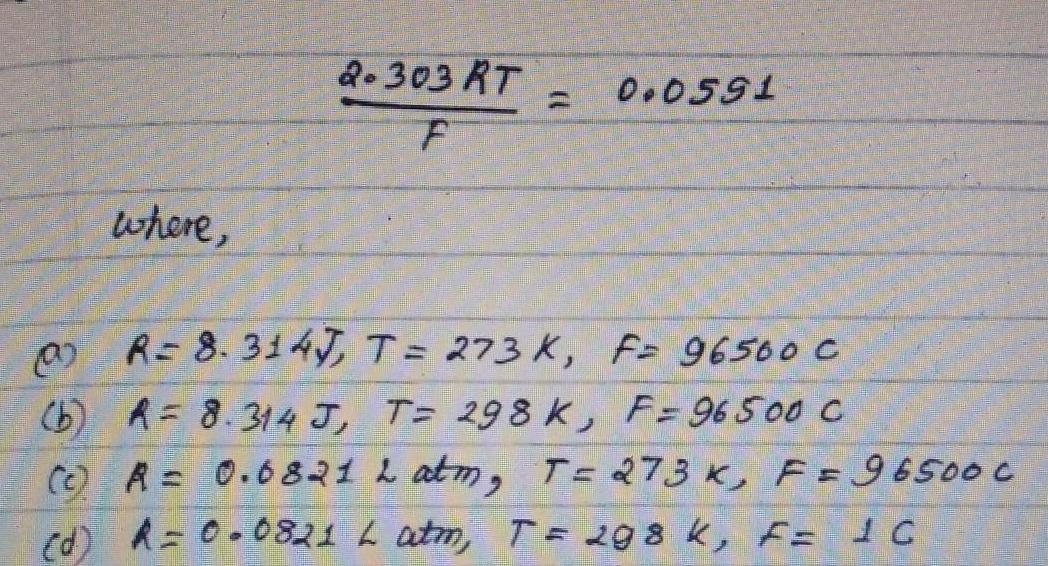Physical Chemistry
Electrochemistry
where 2 303 RT F 0 0591 0 R 8 3147 T 273 K F 96500 C b R 8 314 J T 298 K F 96500 C c R 0 68212 atm T 273 K F 96500 C d R 0 0821 L atm T 298 K F 1 C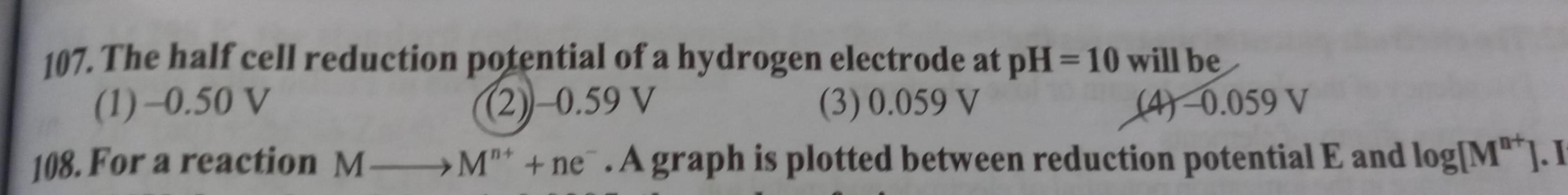Physical Chemistry
Electrochemistry
107 The half cell reduction potential of a hydrogen electrode at pH 10 will be 1 0 50 V 2 0 59 V 3 0 059 V 4 0 059 V 108 For a reaction M M ne A graph is plotted between reduction potential E and log M I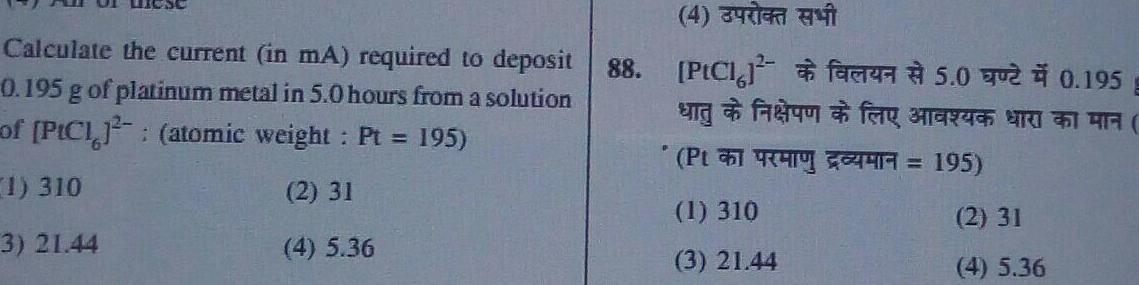Physical Chemistry
Electrochemistry
Calculate the current in mA required to deposit 0 195 g of platinum metal in 5 0 hours from a solution of PICI atomic weight Pt 195 1 310 2 31 3 21 44 4 5 36 88 4 PtCl ungfer 45 0 0 195 for BIERGES BRI Pt 195 1 310 3 21 44 2 31 4 5 36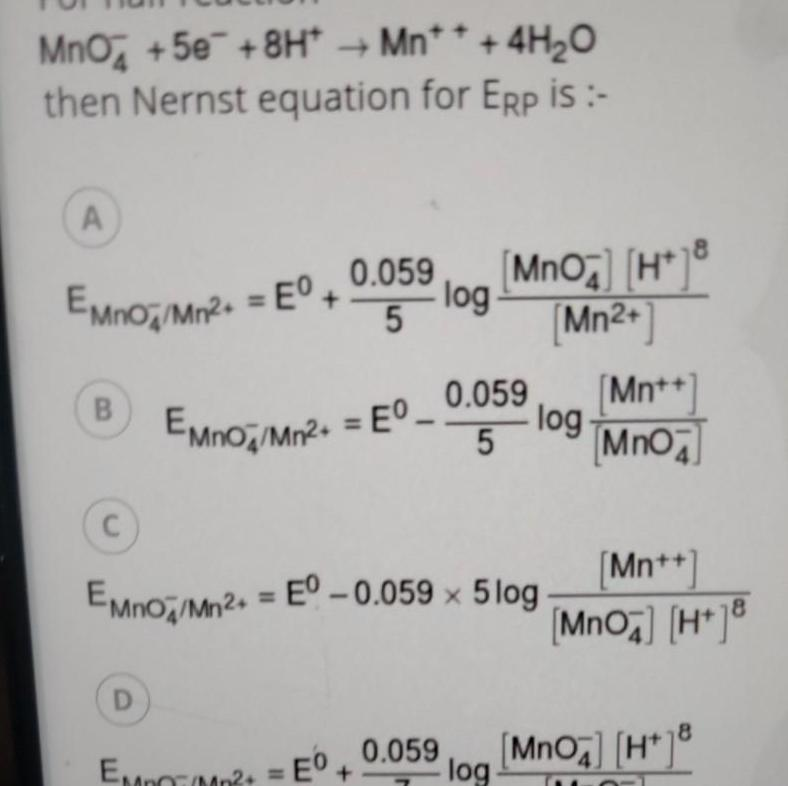Physical Chemistry
Electrochemistry
MnO 5e 8H Mn 4H O then Nernst equation for ERP is A EMnO Mn2 E C 0 059 D B EMno Mn E log MnO4 H 8 Mn 5 log EMPO M2 E EMnO Mn E 0 059 x 5log 0 059 Mn MnO 5 0 059 Mn MnO4 H 8 MnO4 H 8 log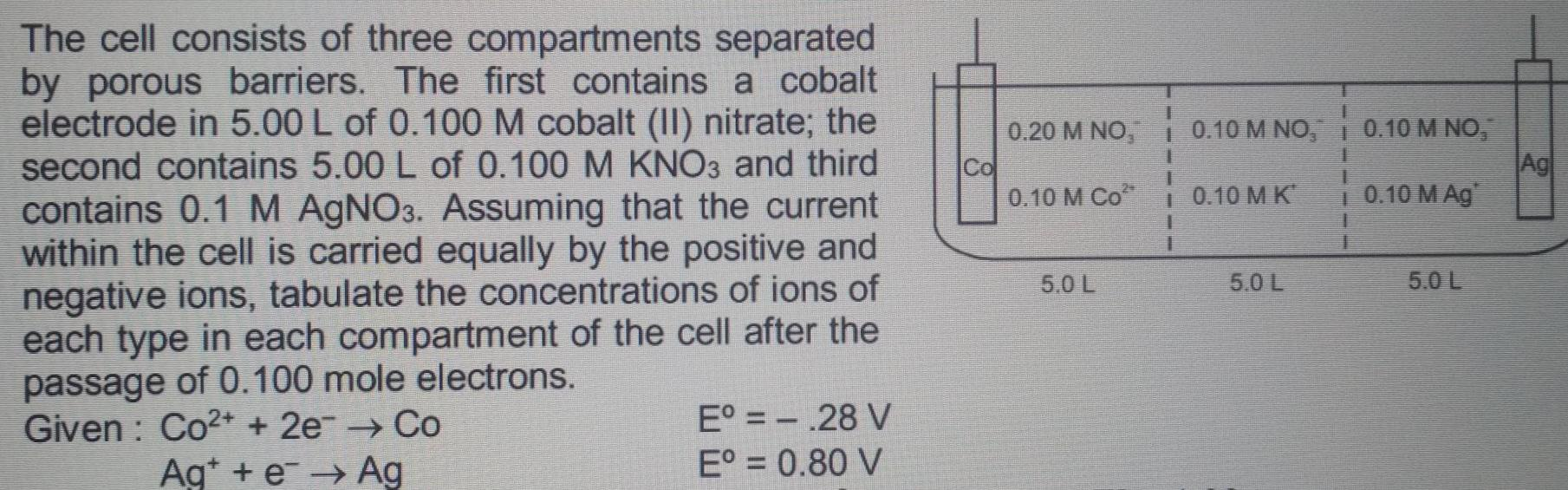Physical Chemistry
Electrochemistry
The cell consists of three compartments separated by porous barriers The first contains a cobalt electrode in 5 00 L of 0 100 M cobalt II nitrate the second contains 5 00 L of 0 100 M KNO3 and third contains 0 1 M AgNO3 Assuming that the current within the cell is carried equally by the positive and negative ions tabulate the concentrations of ions of each type in each compartment of the cell after the passage of 0 100 mole electrons Given Co2 2e Co Age Ag E 28 V E 0 80 V Co 0 20 M NO 0 10 M Co 5 0 L 1 10 10 M NO 1 10 10 M K 1 1 5 0 L 1 0 10 M NO 0 10 M Ag 1 1 5 0 L Ag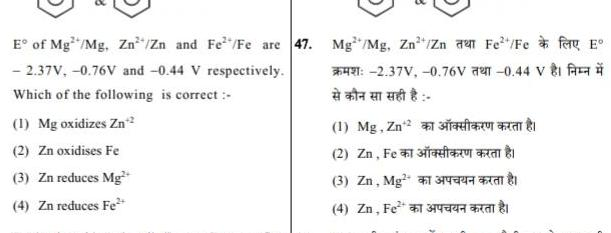Physical Chemistry
Electrochemistry
E of Mg Mg Zn Zn and Fe Fe are 47 2 37V 0 76V and 0 44 V respectively Which of the following is correct 1 Mg oxidizes Zn 2 Zn oxidises Fe 3 Zn reduces Mg 4 Zn reduces Fe Mg Mg Zn Zn e Fe Fefa E 2 37V 0 76V 0 44 V 1 Mg Zn 2 2 Zn Fe 3 3 Zn Mg 4 Zn Fe 1 340 1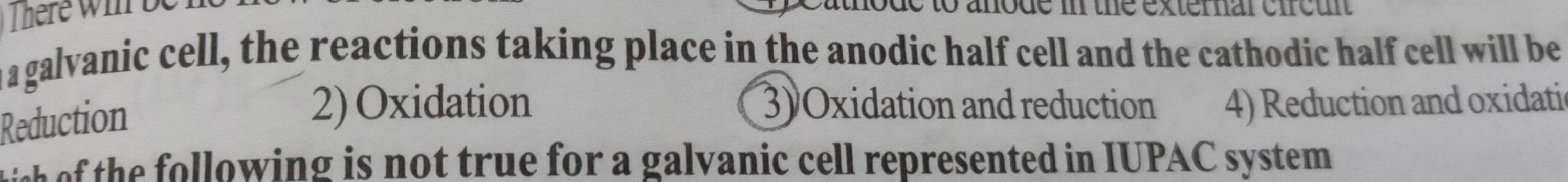Physical Chemistry
Electrochemistry
galvanic cell the reactions taking place in the anodic half cell and the cathodic half cell will be 3 Oxidation and reduction 2 Oxidation 4 Reduction and oxidatio Reduction of the following is not true for a galvanic cell represented in IUPAC system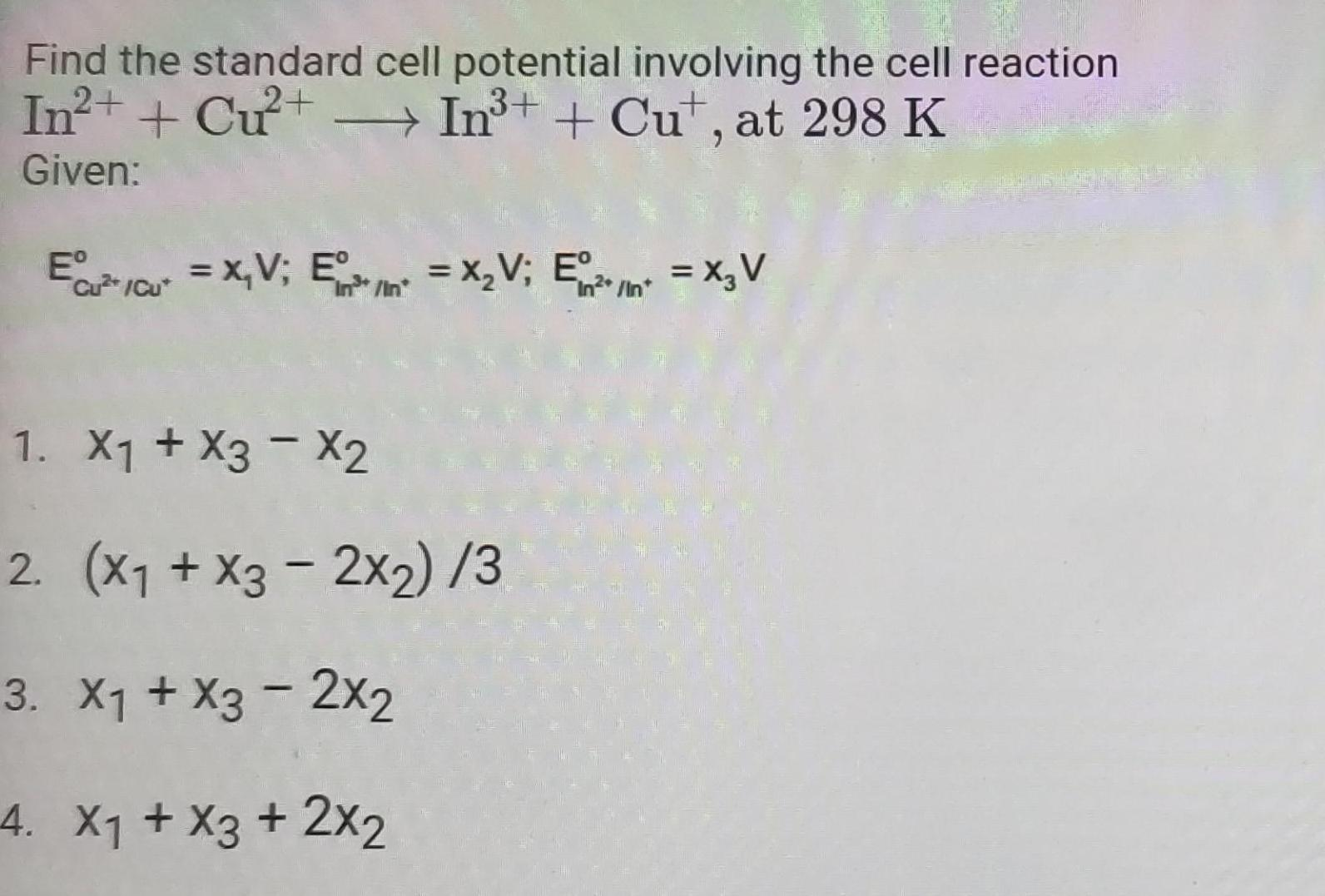Physical Chemistry
Electrochemistry
Find the standard cell potential involving the cell reaction 3 In Cu In Cut at 298 K Given EC Cu X V E X V E X V 1 X X3 X2 2 x x3 2x2 3 3 X1 X3 2x2 4 X X3 2x2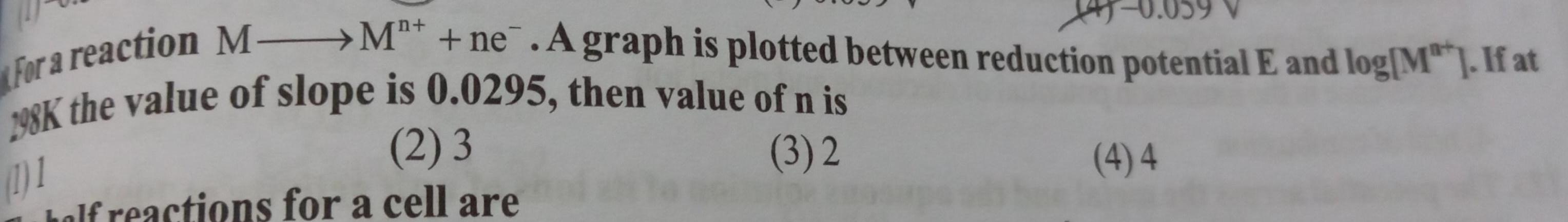Physical Chemistry
Electrochemistry
For a reaction MM ne A graph is plotted between reduction potential E and log M If at 298K the value of slope is 0 0295 then value of n is 3 2 1 1 2 3 holf reactions for a cell are 4 4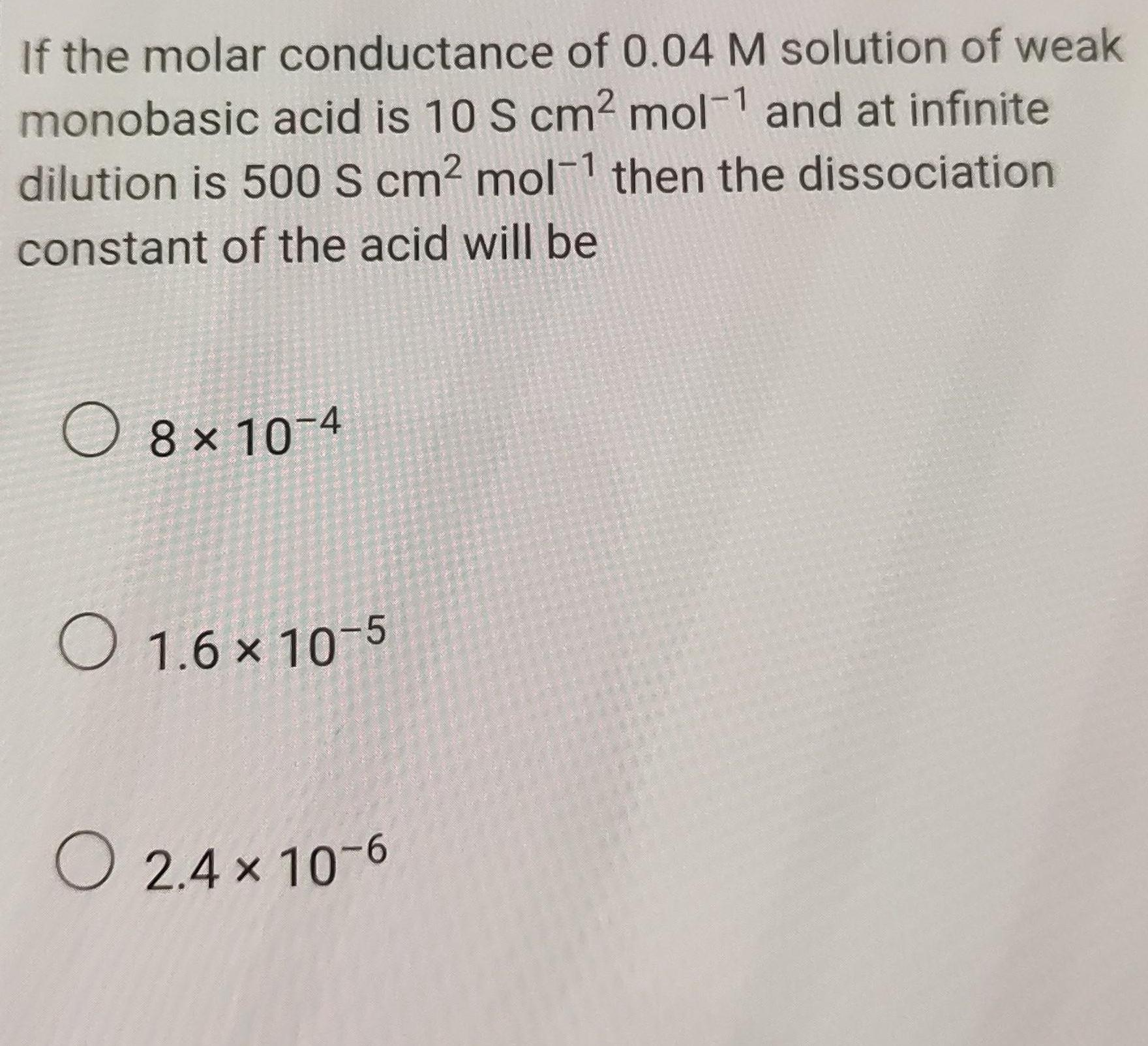Physical Chemistry
Electrochemistry
If the molar conductance of 0 04 M solution of weak monobasic acid is 10 S cm mol 1 and at infinite dilution is 500 S cm mol then the dissociation constant of the acid will be 8x10 4 1 6 10 5 X O 2 4 x 10 6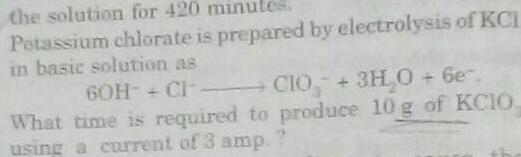Physical Chemistry
Electrochemistry
the solution for 420 minutes Potassium chlorate is prepared by electrolysis of KCI in basic solution as 6OH CI CIO 3H O 6e What time is required to produce 10 g of KCIO using a current of 3 amp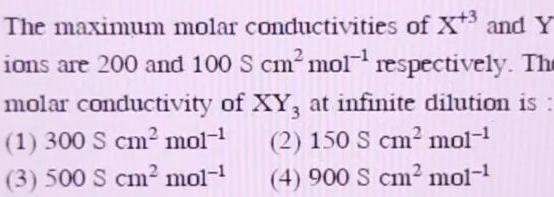Physical Chemistry
Electrochemistry
The maximum molar conductivities of X 3 and Y ions are 200 and 100 S cm mol respectively The molar conductivity of XY3 at infinite dilution is 1 300 S cm mol 2 150 S cm mol 3 500 S cm mol 4 900 S cm mol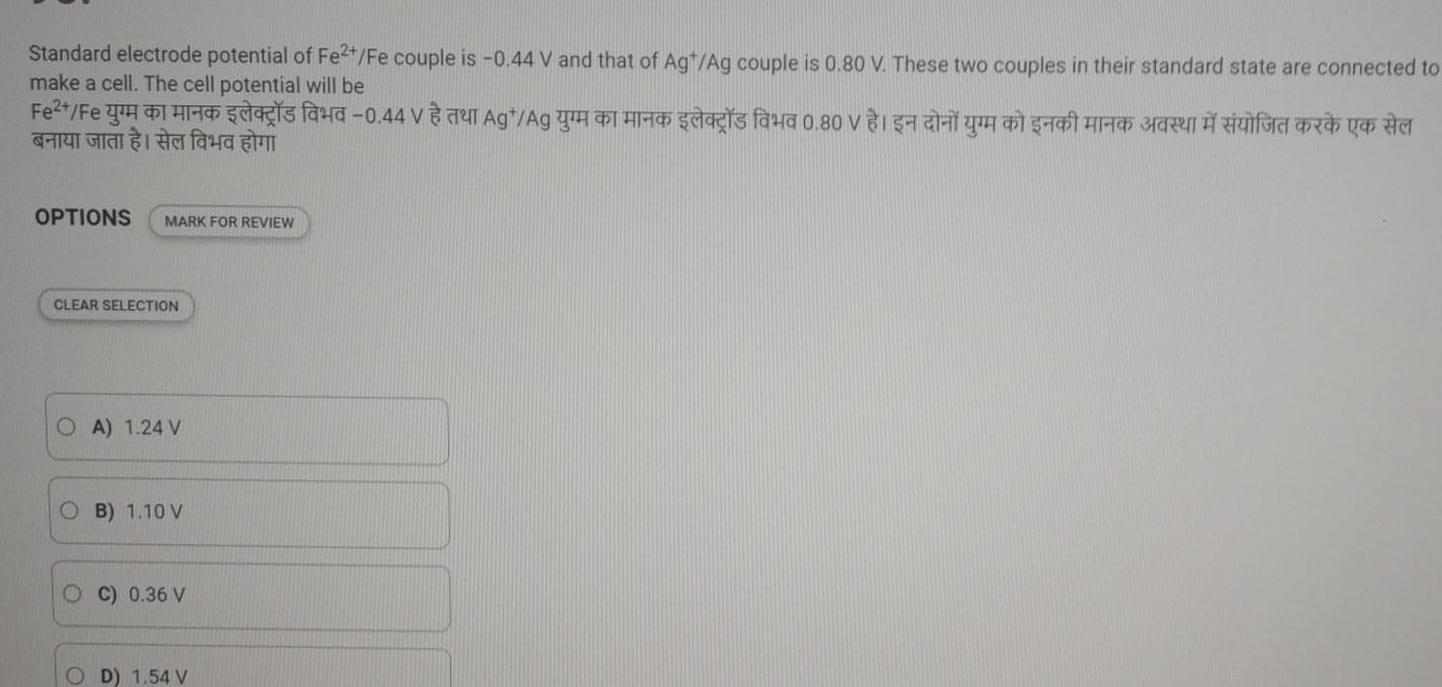Physical Chemistry
Electrochemistry
Standard electrode potential of Fe2 Fe couple is 0 44 V and that of Agt Ag couple is 0 80 V These two couples in their standard state are connected to make a cell The cell potential will be 0 80 G OPTIONS MARK FOR REVIEW CLEAR SELECTION OA 1 24 V OB 1 10 V C 0 36 V OD 1 54 V 0 44 G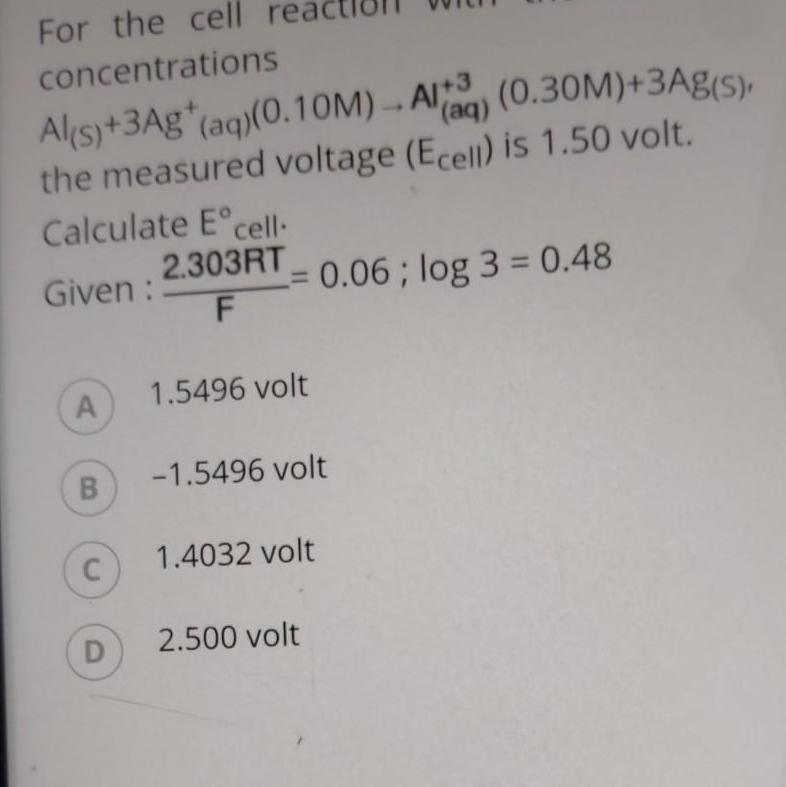Physical Chemistry
Electrochemistry
For the cell concentrations Al s 3Ag aq 0 10M AI 3 0 30M 3Ag s the measured voltage Ecell is 1 50 volt Calculate E cell 2 303RT Given F A B C D 0 06 log 3 0 48 1 5496 volt 1 5496 volt 1 4032 volt 1 2 500 volt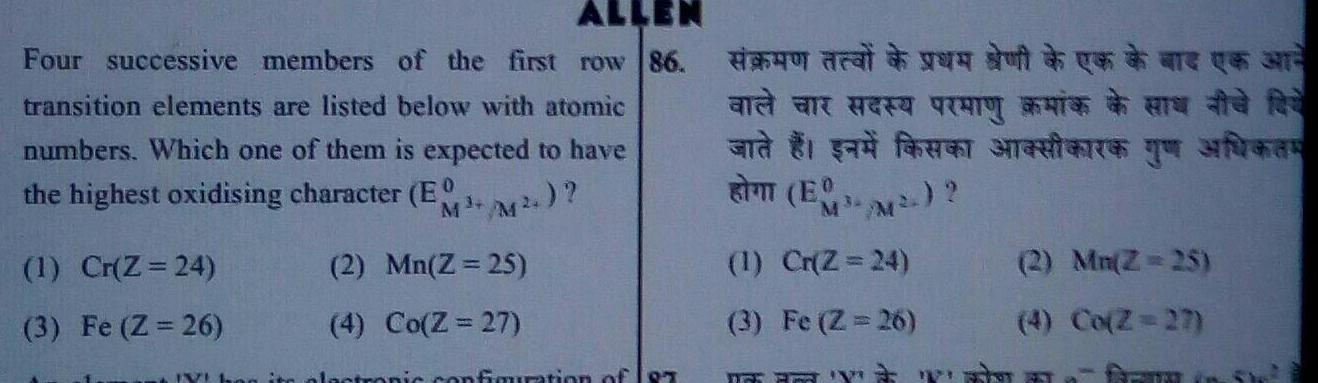Physical Chemistry
Electrochemistry
Four successive members of the first row 86 transition elements are listed below with atomic numbers Which one of them is expected to have the highest oxidising character EO M M 1 Cr Z 24 3 Fe Z 26 2 Mn Z 25 4 Co Z 27 nic configuration of 197 to start an and an ang and g E a fan antare e far 2 1 M 1 Cr Z 24 3 Fe Z 26 Y wi 2 Mn Z 25 4 Co Z 27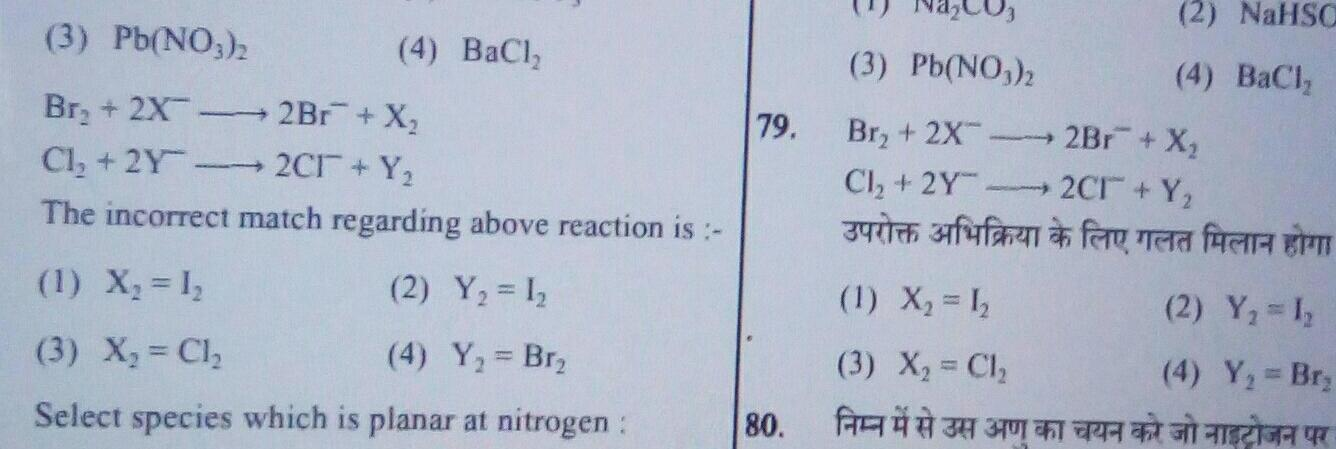Physical Chemistry
Electrochemistry
3 Pb NO3 2 Br 2X 2Br X Cl 2Y 2Cr Y The incorrect match regarding above reaction is 1 X 1 2 Y 1 3 X Cl 4 Y Br Select species which is planar at nitrogen 4 BaCl 79 80 3 Pb NO3 2 Br 2X Cl 2Y uten arfufaen 1 X 1 3 X Cl 2 NaHSC 4 BaCl 2Br X 2Cr Y for a fe 2 Y 1 4 Y Br